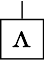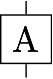## Saturday, May 16, 2009

Knot diagrams aren't the only kind of diagram that can be translated nicely into Haskell monad notation. Other types of diagram include Penrose Spin Networks, many kinds of Feynman Diagram, Penrose Tensor Notation, birdtracks and a type of closely related diagram I hadn't met before: Trace Diagrams.

I encourage readers to check out the Wikipedia page and associated papers on trace diagrams as they give a better tutorial than I could write. My aim here is to show how those diagrams can be translated directly into working code just like with knots.

As usual, this is literate Haskell so I need these lines:

`> {-# LANGUAGE MultiParamTypeClasses,FunctionalDependencies,FlexibleInstances #-}> module Main where> import qualified Data.Map as M> import Control.Monad> infixl 5 .+> infixl 6 .*`

I'll reuse my vector space monad code from before and work in a 3D space with the axes labelled X, Y and Z.
`> data Space = X | Y | Z deriving (Eq,Show,Ord)`

We draw vectors as little boxes with connections emerging from them:Now recall from my knot posts that we represent a diagram with m legs at the top and n legs at the bottom as an expression that takes an m-tuple as input and returns an n-tuple "in the monad" as output.

Vectors can be represented as elements of `V Float Space`, for example:
`> u,v,w :: V Float Space> u = return X .- return Y> v = return X .+ 2.* return Y> w = return Y .- return Z`

I could have emphasised that there are zero inputs at the top by using type signature `() -> V Float Space` instead.

Given two vectors we can form their dot product. The dot product itself is represented by a little u-shaped curve:So the dot product of v and w is drawn as:(The i and j are just so you can see what corresponds to what in the code below. They're not really part of the diagram.)

We can implement the dot product as
`> cup :: (Space,Space) -> V Float ()> cup (i,j) = case (i,j) of>    (X,X) -> return ()>    (Y,Y) -> return ()>    (Z,Z) -> return ()>    otherwise -> 0 .* return ()`

and compute an example using
`> vdotw = do>     i <- v>     j <- w>     cup (i,j)`

We hook up legs of the diagram using corresponding inputs and outputs in the code.

Now consider this diagram:If we attach another vector to the free leg then we get the dot product. So this object is a thing that maps vectors to scalars. Ie. it's a dual vector. So dual vectors are represented by diagrams with a free leg at the top. We can redraw this diagram:In other words, turning a vector v upside down turns it into a dual vector that takes w to the dot product of v and w. Here's some code for making the dual of v.
`> dual :: V Float Space -> Space -> V Float ()> dual v i = do>     j <- v>     cup (i,j)`

We can also consider cross products. These take two vectors as input and output one. So we're looking at a diagram with two legs at the top and one at the bottom. We'll use a bold dot to represent one of these:Here's the implementation:
`> cross :: (Space,Space) -> V Float Space> cross (X,Y) = return Z> cross (Y,Z) = return X> cross (Z,X) = return Y> cross (Y,X) = (-1) .* return Z> cross (Z,Y) = (-1) .* return X> cross (X,Z) = (-1) .* return Y> cross _ = mzero`

We can form a triple product u.(v×w) like this:We can then abstract out the triple product bit that looks like this:Implementing it as:
`> trident :: (Space,Space,Space) -> V Float ()> trident (i,j,k) = do>    l <- cross (i,j)>    cup (l,k)`

Remember that if u, v and w give the rows of a 3x3 matrix, then u.(v×w) is the determinant of that matrix.

We can also define a dot product for dual vectors that we can draw like this:The code looks like this:
`> cap :: () -> V Float (Space,Space)> cap () = return (X,X) .+ return (Y,Y) .+ return (Z,Z)`

We can now combine the two dot products in a diagram like this:We can write that as:
`> cupcap i = do>      (j,k) <- cap ()>      cup (i,j)>      return k`

We'd hope that we could pull this diagram taut and get the identity linear map. If you try applying `cupcap` to X, Y and Z you'll see it has exactly the same effect as `return`, which does indeed represent the identity.

(If you allow me to digress, I'll point out that there's something really deep going on with this almost trivial looking identity. It represents the identity map in the sense that it copies the input i to the output k. Imagine we were dealing with the trivial monad, ie. the one that just wraps values. Then no matter how `cup` and `cap` were implemented it would be impossible for k to be a copy of i. If you follow the flow of information through that code then i disappears into `cup` and k is read from `cap` without it seeing i. If we read from top to bottom we can think of cap as emitting a pair of objects and of cup as absorbing two. There is no way that any information about i can be communicated to k. But in the vector space monad, k can depend on i. As I've mentioned a few times over the years, the universe is described by quantum mechanics which can be described using the vector space monad. Amazingly the above piece of code, or at least something like it, can be physically realised in terms of particles. It describes a process that is fundamentally quantum, and not classical. In fact, Coecke shows that this is a precursor to quantum teleportation in section 3c of this paper. You could also think in terms of information about i being sent back in time through the cap. That's the idea behind this paper on Effective Quantum Time Travel.)

Now we can make a fork by bending down the tines of the cross product:`> fork () = do>    (i,j) <- cap ()>    (k,l) <- cap ()>    m <- cross (j,k)>    return (i,l,m)`

We can write matrices as boxes with a leg for input and a leg for output. Here's an example matrix:`> a :: Space -> V Float Space> a X = 2 .* return X> a Y = return Z> a Z = (-1) .* return Y`

It rotates by 90 degrees around the X axis and scales the X axis by a factor of two.

With the help of our two dot products we can turn a matrix upside-down:The corresponding code is:
`> b :: Space -> V Float Space> b l = do>     (i,j) <- cap ()>     k <- a j>     cup (k,l)>     return i`

Turning a matrix upside down gives its transpose. You'll find that matrix B rotates in the opposite direction to A but still scales by a factor of two.

Surprisingly, 3! times the determinant of a 3x3 matrix A can be represented by this diagram:So we can write a determinant routine as follows:
`> det a = do>     (i,j,k) <- fork ()>     i' <- a i>     j' <- a j>     k' <- a k>     (1/6.0) .* trident (i',j',k')`

(Again I've labelled the diagram so you can easily see what corresponds where in the code.)

I could keep going, but at this point I'll just defer to Elisha Peterson's paper. I hope that I've given you enough clues to be able to translate his diagrams into Haskell code, in effect giving semantics for his syntax. As an exercise, try writing code to compute the adjugate of a 3x3 matrix.

And a reminder: none of the above is intended as production-worthy code for working with 3-vectors. It is intended purely as a way to give a practical realisation of trace diagrams allow people to experimentally investigate their properties and make testable conjectures.

And now comes the library code needed to make the above code work:

`> swap (x,y) = (y,x)> class Num k => VectorSpace k v | v -> k where>    zero :: v>    (.+) :: v -> v -> v>    (.*) :: k -> v -> v>    (.-) :: v -> v -> v>    v1 .- v2 = v1 .+ ((-1).*v2)> data V k a = V { unV :: [(k,a)] }> instance (Num k,Ord a,Show a) => Show (V k a) where>   show (V x) = show (reduce x)> reduce x = filter ((/=0) . fst) \$ fmap swap \$ M.toList \$ M.fromListWith (+) \$ fmap swap \$ x> instance (Ord a,Num k) => Eq (V k a) where>  V x==V y = reduce x==reduce y> instance (Ord a,Num k,Ord k) => Ord (V k a) where>  compare (V x) (V y) = compare (reduce x) (reduce y)> instance Num k => Functor (V k) where>    fmap f (V as) = V \$ map (\(k,a) -> (k,f a)) as> instance Num k => Monad (V k) where>    return a = V [(1,a)]>    x >>= f = join (fmap f x)>        where join x = V \$ concat \$ fmap (uncurry scale) \$ unV \$ fmap unV x>              scale k1 as = map (\(k2,a) -> (k1*k2,a)) as> instance Num r => MonadPlus (V r) where>    mzero = V []>    mplus (V x) (V y) = V (x++y)> instance (Num k,Ord a) => VectorSpace k (V k a) where>    zero = V []>    V x .+ V y = V (x ++ y)>    (.*) k = (>>= (\a -> V [(k,a)]))> e = return :: Num k => a -> V k a> coefficient b (V bs) = maybe 0 id (lookup b (map swap (reduce bs)))`Shin no Noir said...

So far, a nice and mind blowing article (I've read up to the definition of cupcap so far). :)

I just played around with the code and wrote a dot product function using cap:

> dot v w = do
> (i,j) <- cap ()
> v' i
> w' j
> where v' = dual v
> w' = dual w

(Btw, there's a small typo in your digression: "cup and cup" should be "cup and cap".)

Saturday, 23 May, 2009Shin no Noir said...

Btw sigfpe, I was wondering, what do you use to draw these nice diagrams?

Saturday, 23 May, 2009sigfpe said...

Shin no Noir,

If you wait a bit (probably < 1 day) I'll post the LaTeX source code.

Saturday, 23 May, 2009sigfpe said...

I used LaTeX with TikZ to create the diagrams. Here's a link to the source code: http://www.sigfpe.com/trace.tgz You'll need to install TikZ. I used the LaTeX Equation Editor to create the png files: http://evolve.lse.ac.uk/software/EquationEditor/

Despite how good TikZ is, it was a painful process. I kept saying to myself, I'll never write again about something involving diagrams :-)

The author of the paper I cite has written a tutorial on creating exactly this type of diagram: http://elishapeterson.wikidot.com/tikz:diagrams I didn't use it because I wanted to learn TikZ from scratch myself first.

Saturday, 23 May, 2009EBP said...

This is really cool!! Thanks for posting the link to my paper. I always think that trace diagrams should be more widely known.

I've got a more accessible to trace diagrams on the way... will post here when I get it done.

Monday, 31 August, 2009